Floor

The floor area of ​​the room is 31 m2 and has a width of 4.3 m. How many centimeters of circumference measured floor on the map at the scale 1:75?

Result

o =  30.7 cm

Solution:Leave us a comment of example and its solution (i.e. if it is still somewhat unclear...):Be the first to comment!To solve this example are needed these knowledge from mathematics:

Need help calculate sum, simplify or multiply fractions? Try our fraction calculator. Do you want to convert length units?

Next similar examples:

1. Carpet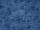The room has rectangle shape with dimensions 4 m × 3.8 m. How many meters of carpet a width of 3 need to buy to cover the entire room?
2. Square to rectangle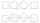What is the ratio of the area of a square of side x to the area of a rectangle of a rectangle of width 2 x and length 3
3. Drawer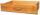The rectangular face of a drawer has a perimeter of 108 cm and a width of 18cm. Find the length and the area of the rectangular face of the drawer.
4. Area of rectangleCalculate the area of rectangle in square meters whose sides have dimensions a = 80dm and b = 160dm.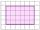Shade the area on the grid that shows 5/8 x 2/4
6. Rectangle vs square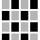The rectangle has dimensions of 13 × 10, square 8 × 8. Which shape has more area and how much above?
7. RectangleThe rectangle area is 182 dm2, its base is 14 dm. How long is the other side? Calculate its perimeter.
8. Garden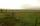The garden has two opposite parallel fences. Their distance is 33.1 m. Lengths in these two fences are 75.5 meters and 49.4 meters. Calculate the area of this garden.
9. Garden plotCalculate how many meters of fence need to fence the square garden with length and width of 22 meters.
10. Rolls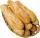Mom bought 13 rolls. Dad ate 3.5 rolls. How many rolls left when Peter yet put two at dinner?
11. A rectangularA rectangular garden 40 m long and 30 m wide is to be fenced with fence posts at each corner. All the other posts will be 5 meters a part. How many posts will be needed to fence in the garden.
12. Package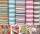The package was 23 meters of textile. The first day sold 12.3 meters. How many meters of textile remained in the package?
13. CakesOn the bowl were a few cakes. Jane ate one-third of them, Dana ate a quarter of those cakes that remained. a) What part (of the original number of cakes) Dana ate? b) At least how many cakes could be (initially) on thebowl?
14. Table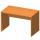Find the circumference of the table, where the long side is 1.28 meters and the short side is 86 cm.
15. Train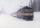The train passes part of the line for 95 minutes at speed 75 km/h. What speed would have to go in order to shorten the driving time of 20 minutes?
16. RectangleCalculate perimeter of the rectangle with sides a=2.4 m and b=1.9 m.
17. Ratio v2Decrease in the ratio 12:16 number 13.2.